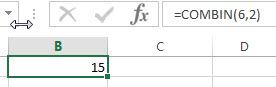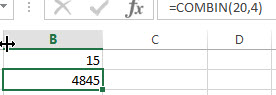# Excel COMBIN Function

This post will guide you how to use Excel COMBIN function with syntax and examples in Microsoft excel.

### Description

The Excel COMBIN function returns the number of combinations for a given number of items. And you can use the COMBIN function to determine the total possible number of groups for a given number of items.

For example, assuming that you have 6 objects(a,b,c,d,e,f) and you can get 15 different combinations of 2 objects , the combinations are as follows:

`ab ac ad ae af bc bd be bf cd ce cf de df ef `

The COMBIN function is a build-in function in Microsoft Excel and it is categorized as a Math and Trigonometry Function.

The COMBIN function in Excel 2016, Excel 2013, Excel 2010, Excel 2007, Excel 2003, Excel XP, Excel 2000, Excel 2011 for Mac.

### Syntax

The syntax of the COMBIN function is as below:

`=COMBIN (number, number_chosen)`

Where the COMBIN function arguments are:

• number – This is a required argument. . The number of items. It must be greater than 0, and it also must be greater than number_chosen argument.
• number_chosen – This is a required argument. The number of combinations that you want to.

Note:

• If either the number argument or the number_chosen argument is less than 0, then the COMBINA function will return the #NUM! Error.
• If any arguments are non-numeric, this function will return #VALUE! Error.
• If any arguments are integer values and will be truncated.
• if you want to calculate the number of combinations for a given number of items (with repetitions), you can use the COMBINA function.

### Excel COMBIN Function Examples

The below examples will show you how to use Excel COMBIN Function to get the number of combinations for a given number objects from a set (in any order).

1# to get the number of combinations for 6 and 2(in any order), enter the following formula in Cell B1.

`=COMBIN(6,2)`2# to get the number of combinations for 20 and 4(in any order), enter the following formula in Cell B2.

`=COMBIN(20,4)`### Related Functions

• Excel COMBINA Function
The Excel COMBINA function returns the number of combinations for a given number of items and it includes repetitions. The syntax of the COMBINA function is as below:=COMBINA(number, number_chosen)…
Related Posts

Excel DVARP Function

This post will guide you how to use Excel DVARP function with syntax and examples in Microsoft excel. Description The Excel DVARP Function will get the variance of a population based on the entire population of numbers in a column ...

Excel DVAR Function

This post will guide you how to use Excel DVAR function with syntax and examples in Microsoft excel. Description The Excel DVAR Function will get the variance of a population based on a sample of numbers in a column in ...

Excel DSUM Function

This post will guide you how to use Excel DSUM function with syntax and examples in Microsoft excel. Description The Excel DSUM Function will add the numbers in a column or database that meets a given criteria. And so you ...

Excel DSTDEVP Function

This post will guide you how to use Excel DSTDEVP function with syntax and examples in Microsoft excel. Description The Excel DSTDEVP returns the standard deviation of a population based on the entire population of numbers in a column or ...

Excel DSTDEV Function

This post will guide you how to use Excel DSTDEV function with syntax and examples in Microsoft excel. Description The Excel DSTDEV returns the standard deviation of a population based on a sample of numbers in a column or database ...

Excel DMIN Function

This post will guide you how to use Excel DMIN function with syntax and examples in Microsoft excel. Description The Excel DMIN returns the minimum value from a database or a column of a list that matches the specified conditions. ...

Excel DPRODUCT Function

This post will guide you how to use Excel DPRODUCT function with syntax and examples in Microsoft excel. Description The Excel DPRODUCT returns the product of values from a set of records that match criteria that you specify. The DPRODUCT ...

Excel XIRR Function

This post will guide you how to use Excel XIRR function with syntax and examples in Microsoft excel. Description The Excel XIRR function returns the internal rate of return for a series of cash flows that is not necessarily periodic. ...

Excel VDB Function

This post will guide you how to use Excel VDB function with syntax and examples in Microsoft excel. Description The Excel VDB function calculates the depreciation of an asset for a specified period based on the double declining balance method. ...

Excel SYD Function

This post will guide you how to use Excel SYD function with syntax and examples in Microsoft excel. Description The Excel SYD function calculates the sum-of-years’digits depreciation of an asset for a specified period. And it will return a numeric ...

Sidebar